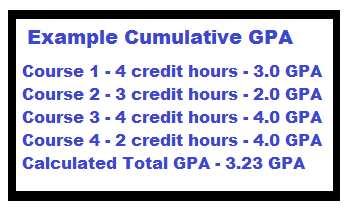Calculate the average weighted GPA of multiple courses with this Weighted Grade Calculator.

Class 1:
--Credit Hours: GPA:

Class 2:
--Credit Hours: GPA:

Class 3:
--Credit Hours: GPA:

Class 4:
--Credit Hours: GPA:

Class 5:
--Credit Hours: GPA:

Class 6:
--Credit Hours: GPA:

Fill in the credit hours and GPA of each class to determine the total weighted grade of the semester. If you took fewer than 6 classes, leave the remaining boxes blank.

### What is a Weighted Grade?

In many college, and some high schools, different classes carry a different "weight" or value of "credit". Many times, classes have a value of "credit hours". You may refer to your semester workload as taking a certain amount of credit hours. Like, "I'm taking 18 credit hours this semester." Those 18 credit hours can be made up of multiple courses that carry a set amount of hours per week of class time. For example, the total of 18 credit hours could be made up from a chemistry class worth 4 credit hours, a geography class worth 3 credit hours, a math class worth 4 credit hours, a literature class worth 3 credit hours and a shop class worth 4 credit hours. What grade you receive in each class is combined and adjusted based on the credit value of each class, to come up with a cumulative weighted grade.

### How to Calculate College GPA, or High School GPA?

To calculate the total weighted GPA of a semester take the following steps:

1. Multiply the GPA and credit hours of each individual class.

2. Take the results of step 1 and add them all together.

3. Divide the total sum from step 2 by the total credit hours taken for the semester.### Weighted Grade Calculator or Cumulative GPA Calculator

Keeping track of your GPA in High School, or College, is one of the most important things a student can do. Knowing how to calculate GPA and how to find cumulative, or weighted GPA based on the amount of credits a class has and the total GPA score of that class can drastically affect a student's final GPA. By utilizing this GPA calculator either as a Weighted Grade Calculator, or as a Cumulative GPA Calculator, you can track your progress and help predict what goals you need to set to achieve your target Grade Point Average.

### How to use the Weighted Grade Calculator

More Resources...

GPA Game
Calculate GPA Video
GPA Tutorial